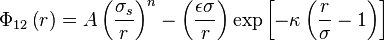2022: SklogWiki celebrates 15 years on-line

Difference between revisions of "Soft sphere attractive Yukawa model"$\Phi_{12}\left( r \right) = A\left( \frac{\sigma_s}{r} \right)^n - \left( \frac{ \epsilon \sigma }{r}\right) \exp \left[- \kappa \left( \frac{r}{\sigma} - 1 \right) \right]$